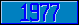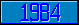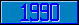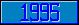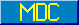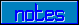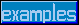## \$%LOWER

### M[UMPS] by Example

```
16-Feb–1996, 16:54:35

Routine Save for Library function %LOWER^STRING

;

; The code below was approved in document X11/1998–21

;

LOWER(A,CHARMOD) NEW lo,up,x,y

SET x=\$GET(CHARMOD)

SET lo="abcdefghijklmnopqrstuvwxyz"

SET up="ABCDEFGHIJKLMNOPQRSTUVWXYZ"

IF x?1"^"1E.E DO

. SET x=\$EXTRACT(x,2,\$LENGTH(x))

. IF x?1"|".E DO

. . SET x=\$REVERSE(\$EXTRACT(x,2,\$LENGTH(x)))

. . SET y=\$REVERSE(\$PIECE(x,"|",2,\$LENGTH(x)+2))

. . SET x=\$REVERSE(\$PIECE(x,"|",1))

. . SET x=\$GET(^|y|\$GLOBAL(x,"CHARACTER"))

. . QUIT

. ELSE  SET x=\$GET(^\$GLOBAL(x,"CHARACTER"))

. QUIT

IF x="" SET x=^\$JOB(\$JOB,"CHARACTER")

SET x=\$GET(^\$CHARACTER(x,"LOWER"))

IF x="" QUIT \$TRANSLATE(A,up,lo)

SET @("x="_x_"(A)")

QUIT x

; ===

;

;

```